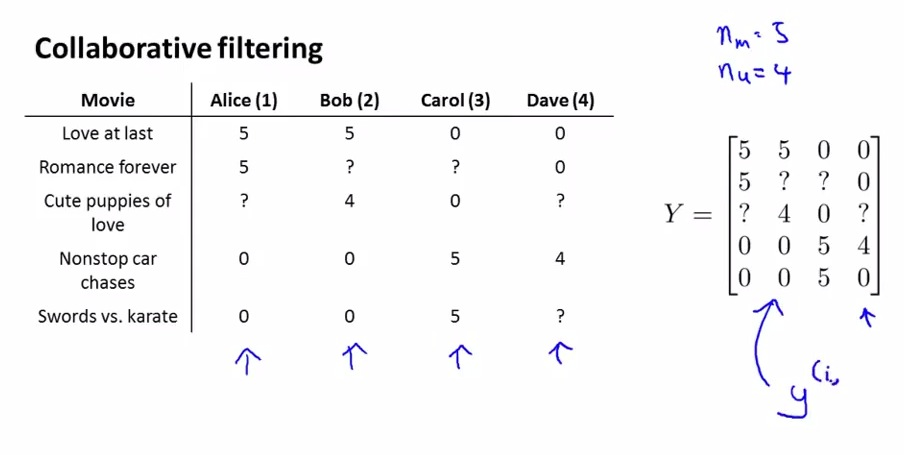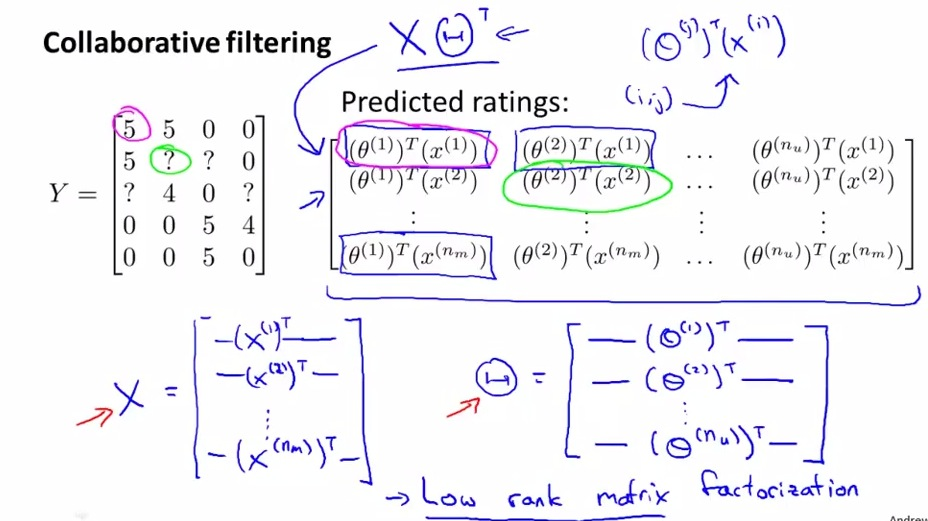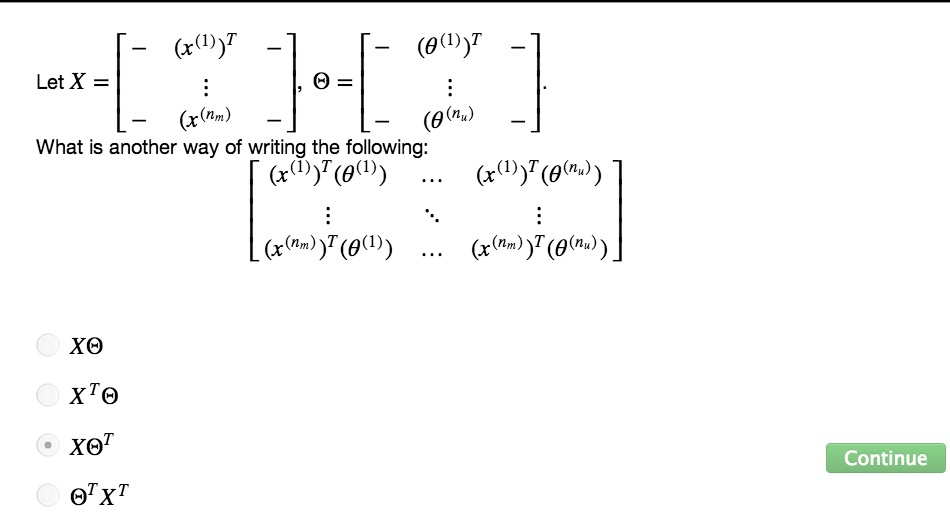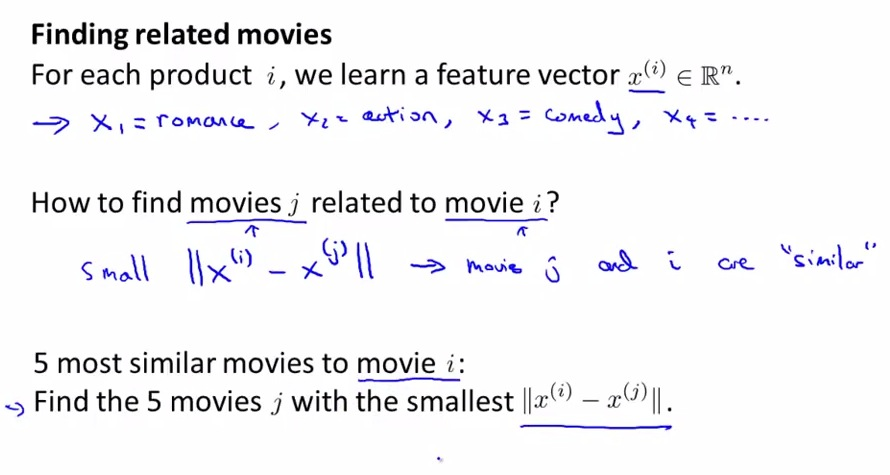• in the last video, we talked about Collaboration Filtering Algorithm
• This video, we talked about how's to do vectorization about Collaboration Filtering Algorithm
• Moreover,extending its benefit, which is find the similarity about other product that user purchased, and recommend it• We already found table example above. Its telling us about the user's rating about each of the movie.
• What is this about, it's the same matrix Y. where y(i,j) is how user j rating about the movie i
• Remember, that the row of matrix Y is the movie, and the column is rating of the users.• Now, we are going to vectorize the prediction implementation about matrix Y. which is y(i,j) is the matrix of user j rating about movie i
• If we see, the predicted ratings,we want to stack all the prediction computation which is done by matrix Y
• What we can do, first observe the matrix element of x and big thetas
• First we know that xi is a feature vector, and we want to make xi vector as one row. So we transpose the matrix xi
• theta also a vector. which is the rating of users based on all movies. Because we want thetai as one row, we also transpose thetas
• For both of all the element of matrix x and theta, we transpose each element, then we want to stack it into big matrix, X and big Theta
• Next to satisfy the calculation about user's prediction ratings, we use X mult bigTheta(t)
• Among the Linear Algebra Expert, this is what called Low Rank Matrix Factorization• Now that we know all the feature in each of the product, we may want to know relatedness between them
• It's often difficult as it's more like human understanding about each product, but it certainly plaussible
• What might be the case in movie examples, is suppose the users purchase some products, and we may want to search of other similar products to recommend to them
• This is reasonable as we want users to keep engaging and purchasing our product
• So what we do is, we are trying to find the smallest distance about other products that user purchased, the smaller the distance is, then the two products is more related
• With this, we can find 5 of the smallest distance, and recommend 5 of most similar movies that we can recommend to the users

• By now, we already know about the vectorized implementation of Collaboration Filtering algoritm
• And we also learn how to use features of the movies, and find similar movies to recommend to users.